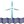# DC Transistor AmplifierElectronic Circuits wrote 10/16/2020 at 00:01 • 2 min read • Like

The only difference between two amplifiers is the difference between Rc1a and Rc1b resistors. Those resistors ensure that the amplifier output is not random when the Q2a and Q2b transistors are disconnected. This occurs when the Q1a or Q1b collect voltages are equal to the power supply voltage. The Rc1a and Rc1b resistors keep the Q2a and Q2b transistors OFF.

If you would think that Rc1a and Rc1b will have no affect on the output value, then the simulations will show how wrong you are. Rc1a is 10 times more than Rb2a in value. If we do the calculations for the Q1a transistor load then you will find that Rc1a will increase the load and the Q1a transistor, increase the collector current and reducing the load resistance. The Rc1b value that is 100 times more than Rb2b resistor will have less influence on the amplifier gain.

We are assuming that both transistors are biased at half the power supply voltage.

The Q1a load resistance equals to:

RcA = Rc1a || (Rb2a + Beta*re)

= 10,000 ohms || (1,000 ohms + 100 * 0.026 / IeA)

IeA = ((Vs / 2 - Vbe2) / Rb2a + Vs / 2 / Rc1a) / Beta * (Beta + 1)

IeA = 0.0009595 = 0.9595 mA

= 10,000 ohms || (1,000 ohms + 2,709.74465868 ohms)

= 2,705.91812688 ohms

The Q1b load resistance equals to:

RcB = Rc1a || (Rb2a + Beta*re)

= 100,000 ohms || (1,000 ohms + 100 * 0.026 / IeB)

IeB = ((Vs / 2 - Vbe2) / Rb2b + Vs / 2 / Rc1b) / Beta * (Beta + 1)

IeB = 0.00082315 = 0.82315 mA

= 100,000 ohms || (1,000 ohms + 3,158.5980684 ohms)

= 3,992.56340381 ohms

The output voltage is the product (multiplication) of collector resistors (Rc1a or Rc1b) and collector current (Ic1a or Ic1b). Thus increasing the load resistances (Rc1a or Rc1b) might increase the amplifier gain. However, increasing the load resistances (Rc1a or Rc1b) will also reduce the biasing current (Ib1a or Ib1b) and thus the transistor current gain as well that will reduce the collector current (Ic1a or Ic1b).

Simulations

PSpice simulations show the difference in output voltages:

The first amplifier has a higher voltage gain. However, this could be due to current gain differences. By changing the current gain characteristics of each transistor you might be able to increase the current gain of the second amplifier.

## Discussions